# tensorflow + dalex = :) , or how to explain a TensorFlow model

Having a machine learning model that generates interesting predictions is one thing. Understanding why it makes these predictions is another. For a tensorflow predictive model, it can be straightforward and convenient develop an explainable AI by leveraging the dalex Python package.

By Hubert Baniecki, Research Software Engineer at MI2DataLab.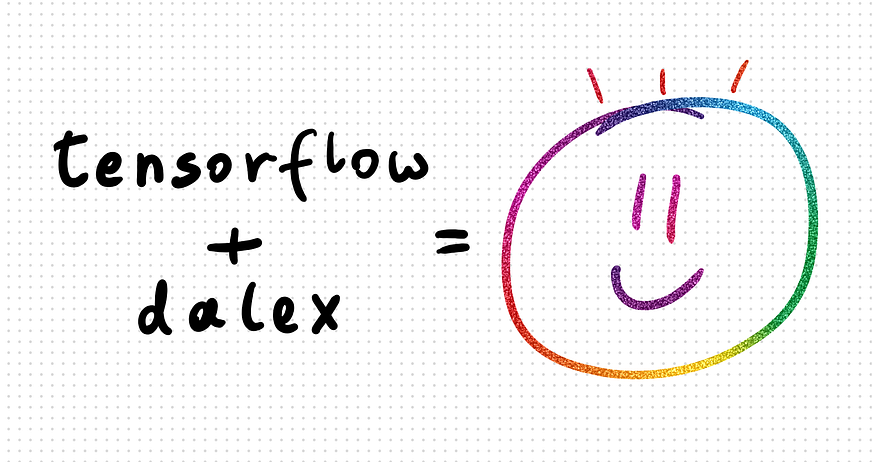I will showcase how straightforward and convenient it is to explain a tensorflow predictive model using the dalex Python package. The introduction to this topic can be found in Explanatory Model Analysis: Explore, Explain, and Examine Predictive Models.

For this example, we will use the data from the World Happiness Report and predict the happiness scored according to economic production, social support, etc., for any given country.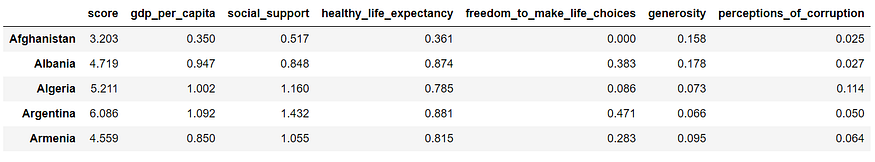Data from the World Happiness Report (Kaggle.com).

Let’s first train the basic tensorflow model incorporating the experimental normalization layer for a better fit.

The next step is to create a dalex Explainer object, which takes model and data as input.

Now, we are ready to explain the model using various methods: model level methods explain the global behavior, while predict level methods focus locally on a single observation from the data. We can start by evaluating model performance.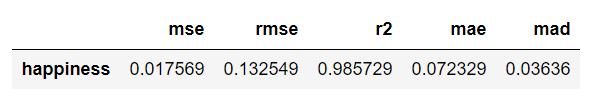Model performance for the happiness regression task.

Which features are the most important? Let’s compare the two methods, one of which is implemented in the shap package.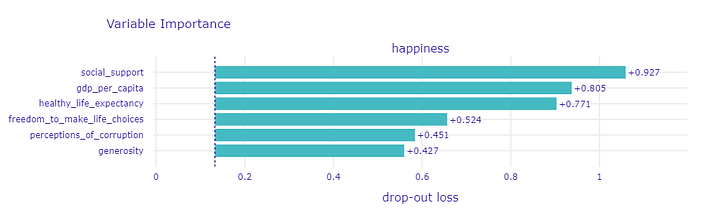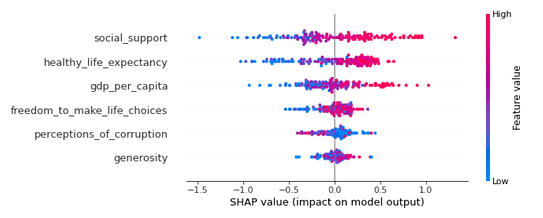Comparison of the two Feature Importance methods, one of which is implemented in the shap package.

What are the continuous relationships between variables and predictions? We use Partial Dependence profiles, which point out that not always the more, the better.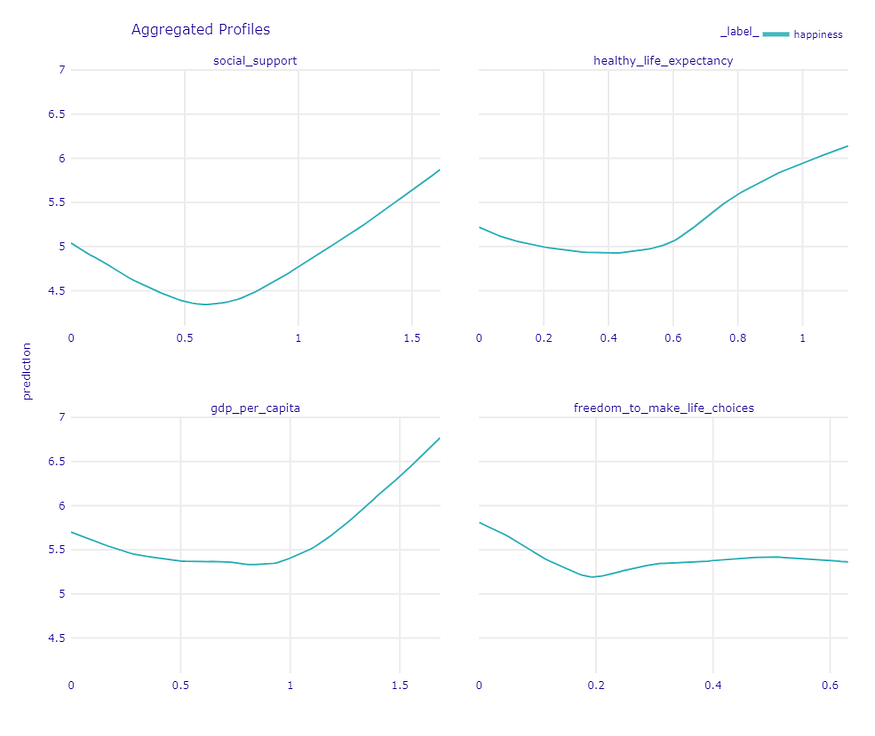Partial Dependence profiles display the continuous relationships between variables and predictions.

What about the residuals? These plots are useful to visualize where the model is wrong.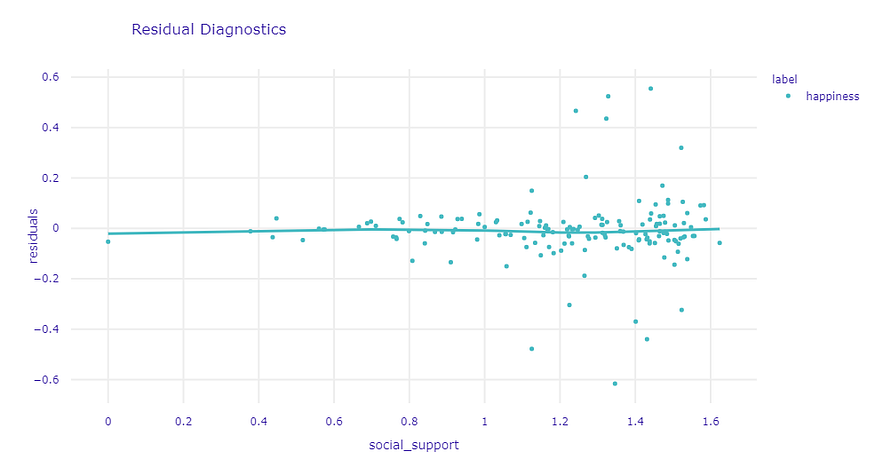Residual diagnostics can help assess weaknesses in our model.

One can be more curious about the variable attributions for a specific country,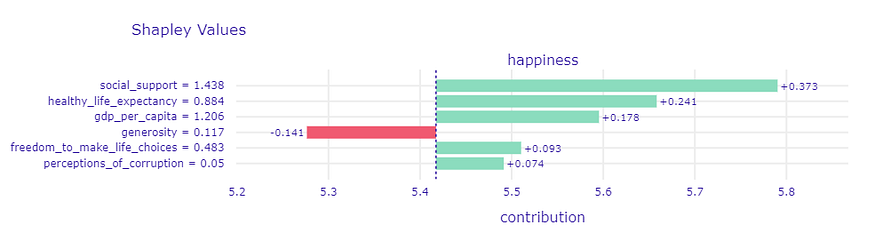Variable attributions for Poland.

or several countries to compare the results.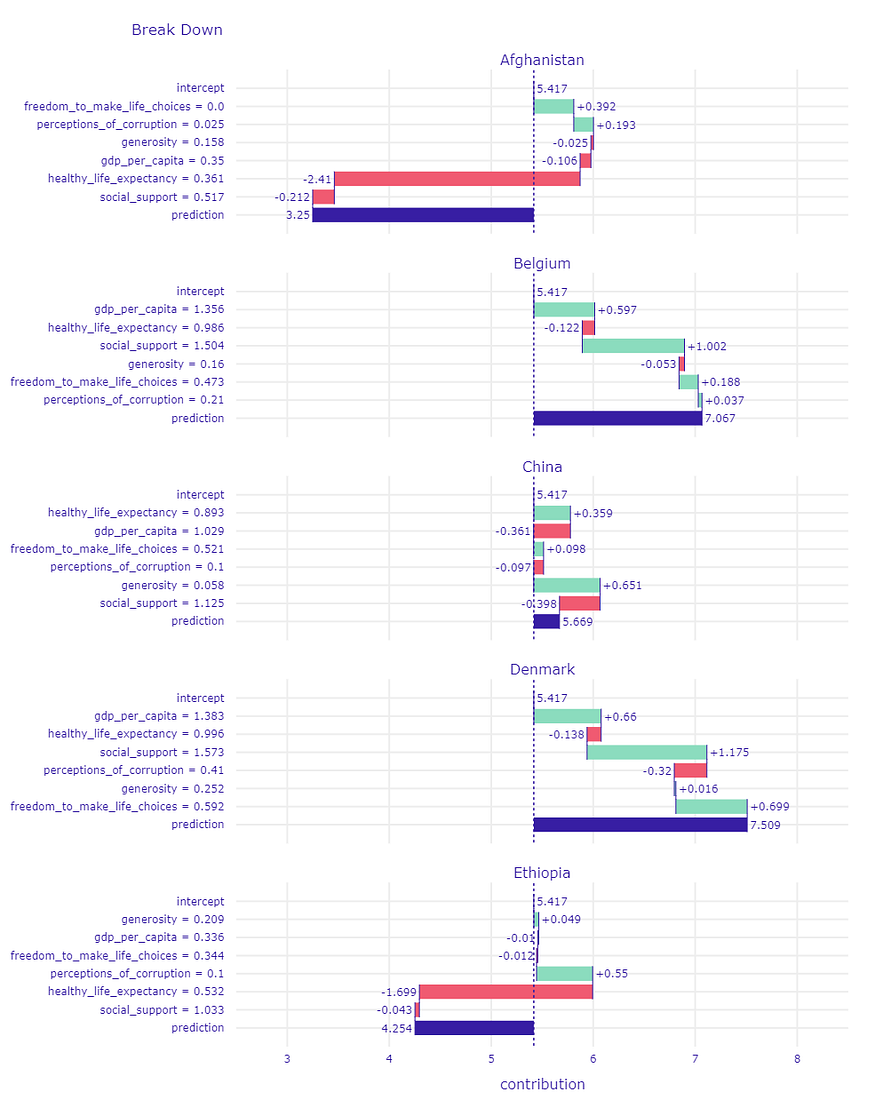Variable attributions for multiple countries.

Should you be interested in surrogate approximation, there is a possibility to produce the lime package explanations using the unified interface.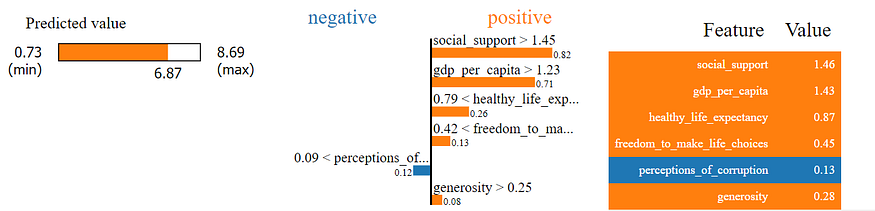Surrogate approximation - an explanation from the lime package.

Finally, if an interpretable model is needed, we can approximate the black-box with an easy-to-understand decision tree.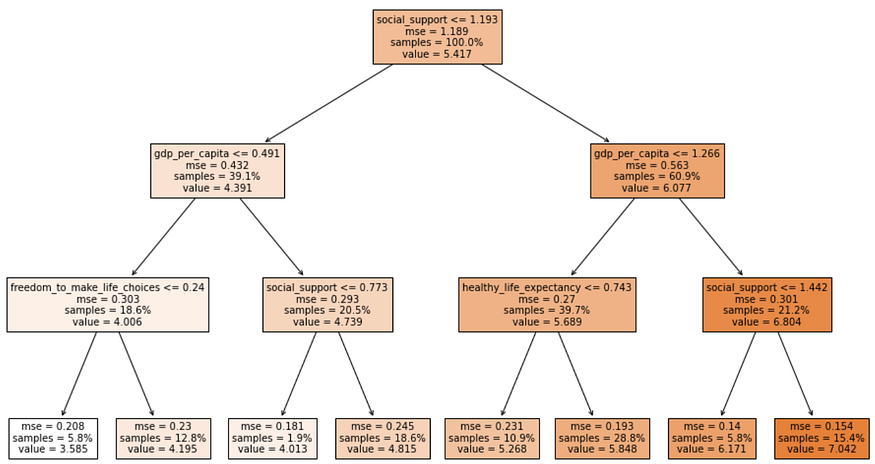Decision tree trained on predicted values of the black-box model.

I hope that this journey brought you some happiness as it is accessible and user-friendly to explain predictive models nowadays. Of course, there are more explanations, results, and plots in the dalex toolkit. We prepared various resources listed in the package README .

The code for this piece is available at http://dalex.drwhy.ai/python-dalex-tensorflow.html.

```pip install tensorflow dalex shap statsmodels lime scikit-learn
```

:)

Original. Reposted with permission.

Bio: Hubert Baniecki is a Research Software Engineer, developing R & Python tools for Explainable AI, and researching ML in the context of interpretability and human-model interaction.

Related:

By subscribing you accept KDnuggets Privacy Policy

By subscribing you accept KDnuggets Privacy Policy

By subscribing you accept KDnuggets Privacy Policy